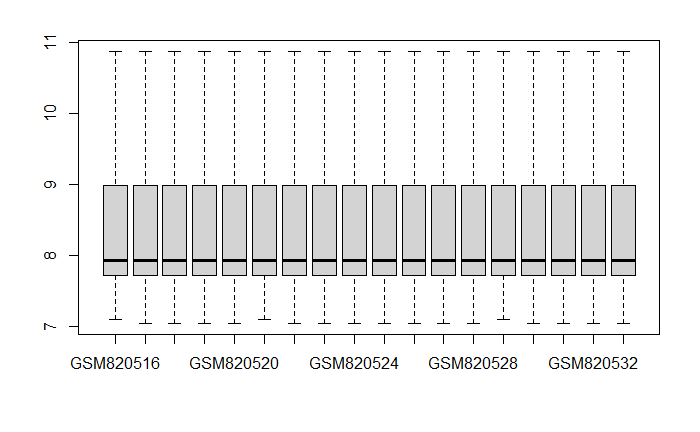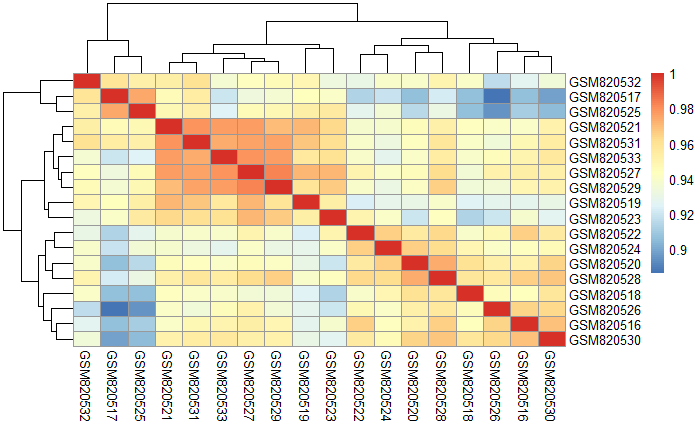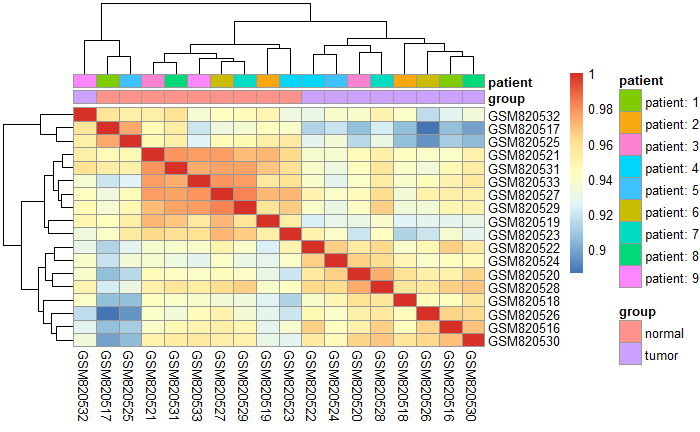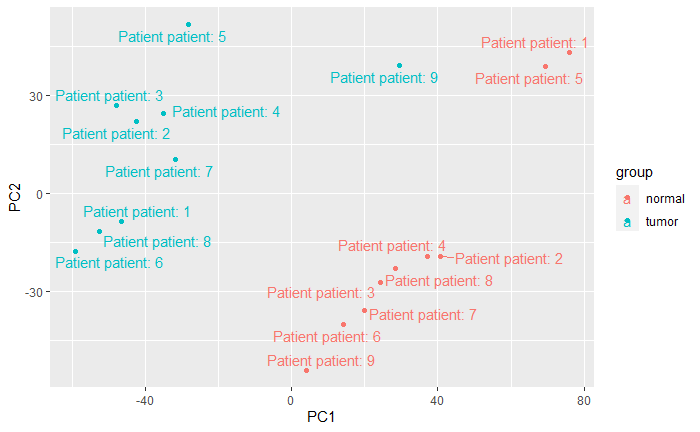# Introduction

In this tutorial we will demonstrate how to download data from Gene Expression Omnibus directly into R. Once loaded, we will perform some quality assessment, differential expression and downstream analysis such as clustering.

We will illustrate the main steps in the workflow. However, some steps may need adjusted for your particular analysis (e.g. changing the model for the differential expression).

You will need to install the following packages before starting:-

``````install.packages("BiocManager")
install.packages("forcats")
install.packages("stringr")
install.packages("ggplot2")
install.packages("ggrepel")
install.packages("tidyr")
install.packages("survminer")
BiocManager::install("GEOquery")
BiocManager::install("limma")
BiocManager::install("pheatmap")
BiocManager::install("org.Hs.eg.db")``````

You will also need to be familiar with our introductory materials on the `ggplot2` and `dplyr` packages

https://sbc.shef.ac.uk/workshops/2020-03-03-r/crash-course.nb.html#dealing_with_data

# Importing the data

The data from this experiment comprises nine paired tumor/normal colon tissues on Illumina HT12_v3 gene expression Beadchips. We will assume that you already know the accession number (GSE….) for the dataset that you want to download.

The function to download a GEO dataset is `getGEO` from the `GEOquery` package. You have to specify the ID of the dataset that you want. To download your own data, replace `GSE33126` with the ID that you’re interested in.

``````library(GEOquery)
## change my_id to be the dataset that you want.
my_id <- "GSE33126"
gse <- getGEO(my_id)``````
``````Found 1 file(s)
GSE33126_series_matrix.txt.gz
trying URL 'https://ftp.ncbi.nlm.nih.gov/geo/series/GSE33nnn/GSE33126/matrix/GSE33126_series_matrix.txt.gz'
Content type 'application/x-gzip' length 3658664 bytes (3.5 MB)

Parsed with column specification:
cols(
ID_REF = col_character(),
GSM820516 = col_double(),
GSM820517 = col_double(),
GSM820518 = col_double(),
GSM820519 = col_double(),
GSM820520 = col_double(),
GSM820521 = col_double(),
GSM820522 = col_double(),
GSM820523 = col_double(),
GSM820524 = col_double(),
GSM820525 = col_double(),
GSM820526 = col_double(),
GSM820527 = col_double(),
GSM820528 = col_double(),
GSM820529 = col_double(),
GSM820530 = col_double(),
GSM820531 = col_double(),
GSM820532 = col_double(),
GSM820533 = col_double()
)
File stored at:
C:\Users\mjdun\AppData\Local\Temp\Rtmp6fVGF5/GPL6947.soft``````

Some datasets on GEO may be derived from different microarray platforms. Therefore the object `gse` is a list of different datasets. You can find out how many were used by checking the length of the `gse` object. Usually there will only be one platform and the dataset we want to analyse will be the first object in the list (`gse[]`).

``````## check how many platforms used
length(gse)``````
`` 1``
``````gse <- gse[]
gse``````
``````ExpressionSet (storageMode: lockedEnvironment)
assayData: 48803 features, 18 samples
element names: exprs
protocolData: none
phenoData
sampleNames: GSM820516 GSM820517 ... GSM820533 (18 total)
varLabels: title geo_accession ... tissue:ch1 (34 total)
featureData
featureNames: ILMN_1343291 ILMN_1343295 ... ILMN_2416019 (48803 total)
fvarLabels: ID nuID ... GB_ACC (30 total)
experimentData: use 'experimentData(object)'
pubMedIds: 23028787
Annotation: GPL6947 ``````
``````## if more than one dataset is present, you can analyse the other dataset by changing the number inside the [[...]]
## e.g. gse <- gse[]``````
``````pData(gse) ## print the sample information
fData(gse) ## print the gene annotation
exprs(gse) ## print the expression data``````

# Check the normalisation and scales used

For visualisation and statistical analysis, we will inspect the data to discover what scale the data are presented in. The methods we will use assume the data are on a log\(_2\) scale; typically in the range of 0 to 16.

The `exprs` function can retrieve the expression values as a data frame; with one column per-sample and one row per-gene.

The `summary` function can then be used to print the distributions.

``````## exprs get the expression levels as a data frame and get the distribution
summary(exprs(gse))``````
``````   GSM820516         GSM820517         GSM820518         GSM820519         GSM820520         GSM820521         GSM820522
Min.   :  137.4   Min.   :  132.6   Min.   :  132.6   Min.   :  132.6   Min.   :  132.6   Min.   :  137.4   Min.   :  132.6
1st Qu.:  212.1   1st Qu.:  212.1   1st Qu.:  212.0   1st Qu.:  212.1   1st Qu.:  212.0   1st Qu.:  212.1   1st Qu.:  212.1
Median :  244.9   Median :  245.0   Median :  244.9   Median :  244.9   Median :  244.9   Median :  244.9   Median :  244.9
Mean   : 1247.1   Mean   : 1247.1   Mean   : 1247.0   Mean   : 1247.1   Mean   : 1247.1   Mean   : 1247.1   Mean   : 1247.1
3rd Qu.:  508.2   3rd Qu.:  508.2   3rd Qu.:  508.0   3rd Qu.:  508.2   3rd Qu.:  508.2   3rd Qu.:  508.2   3rd Qu.:  508.2
Max.   :71775.3   Max.   :71775.3   Max.   :71775.3   Max.   :71775.3   Max.   :71775.3   Max.   :71775.3   Max.   :71775.3
GSM820523         GSM820524         GSM820525         GSM820526         GSM820527         GSM820528         GSM820529
Min.   :  132.6   Min.   :  132.6   Min.   :  132.6   Min.   :  132.6   Min.   :  132.6   Min.   :  132.6   Min.   :  137.4
1st Qu.:  212.1   1st Qu.:  212.1   1st Qu.:  212.1   1st Qu.:  212.0   1st Qu.:  212.1   1st Qu.:  212.1   1st Qu.:  212.1
Median :  244.9   Median :  245.0   Median :  245.0   Median :  244.9   Median :  245.0   Median :  245.0   Median :  245.0
Mean   : 1247.1   Mean   : 1247.2   Mean   : 1247.2   Mean   : 1247.1   Mean   : 1247.2   Mean   : 1247.1   Mean   : 1247.1
3rd Qu.:  508.2   3rd Qu.:  508.2   3rd Qu.:  508.3   3rd Qu.:  508.3   3rd Qu.:  508.2   3rd Qu.:  508.3   3rd Qu.:  508.2
Max.   :71775.3   Max.   :71775.3   Max.   :71775.3   Max.   :71775.3   Max.   :71775.3   Max.   :71775.3   Max.   :71775.3
GSM820530         GSM820531         GSM820532         GSM820533
Min.   :  132.6   Min.   :  132.6   Min.   :  132.6   Min.   :  132.6
1st Qu.:  212.1   1st Qu.:  212.1   1st Qu.:  212.1   1st Qu.:  212.1
Median :  244.9   Median :  244.9   Median :  245.0   Median :  245.0
Mean   : 1247.0   Mean   : 1247.1   Mean   : 1247.1   Mean   : 1247.1
3rd Qu.:  507.7   3rd Qu.:  507.9   3rd Qu.:  508.1   3rd Qu.:  508.0
Max.   :71775.3   Max.   :71775.3   Max.   :71775.3   Max.   :71775.3  ``````

From this output we clearly see that the values go beyond 16, so we will need to perform a \(log_2\) transformation. A `boxplot` can also be generated to see if the data have been normalised. If so, the distributions of each sample should be highly similar.

``````exprs(gse) <- log2(exprs(gse))
boxplot(exprs(gse),outline=FALSE)``````# Inspect the clinical variables

Data submitted to GEO contain sample labels assigned by the experimenters, and some information about the processing protocol. All these data can be extracted by the `pData` function.

For your own data, you will have to decide which columns will be useful in the analysis. This will include the column giving the main comparison(s) of interest and any potential confounding factors. In this particular dataset it looks like `source_name_ch1` and `characteristics_ch1.1`.

We can use the `select` function from `dplyr` to display just these columns of interest. At this stage it will also be useful to rename the columns to something more convenient using the `rename` function.

``library(dplyr)``
``````
Attaching package: 㤼㸱dplyr㤼㸲

The following object is masked from 㤼㸱package:Biobase㤼㸲:

combine

The following objects are masked from 㤼㸱package:BiocGenerics㤼㸲:

combine, intersect, setdiff, union

The following objects are masked from 㤼㸱package:stats㤼㸲:

filter, lag

The following objects are masked from 㤼㸱package:base㤼㸲:

intersect, setdiff, setequal, union``````
``````sampleInfo <- pData(gse)
sampleInfo``````
``````
## source_name_ch1 and characteristics_ch1.1 seem to contain factors we might need for the analysis. Let's pick just those columns

sampleInfo <- select(sampleInfo, source_name_ch1,characteristics_ch1.1)

## Optionally, rename to more convenient column names
sampleInfo <- rename(sampleInfo,group = source_name_ch1, patient=characteristics_ch1.1)``````

Our sample information is therefore:-

``sampleInfo``

# Sample clustering and Principal Components Analysis

Unsupervised analysis is a good way to get an understanding of the sources of variation in the data. It can also identify potential outlier samples.

The function `cor` can calculate the correlation (on scale 0 - 1) in a pairwise fashion between all samples. This can be then visualised on a heatmap. Among the many options for creating heatmaps in R, the `pheatmap` library is one of the more popular ones. The only argument it requires is a matrix of numerical values (such as the correlation matrix).

``````library(pheatmap)
## argument use="c" stops an error if there are any missing data points

corMatrix <- cor(exprs(gse),use="c")
pheatmap(corMatrix)                ``````We can incorporate sample information onto the plot to try and understand the clustering. We have already created such a data frame previously (`sampleInfo`). However, we need to take care that the rownames of these data match the columns of the correlation matrix.

``````## Print the rownames of the sample information and check it matches the correlation matrix
rownames(sampleInfo)``````
``````  "GSM820516" "GSM820517" "GSM820518" "GSM820519" "GSM820520" "GSM820521" "GSM820522" "GSM820523" "GSM820524" "GSM820525" "GSM820526"
 "GSM820527" "GSM820528" "GSM820529" "GSM820530" "GSM820531" "GSM820532" "GSM820533"``````
``colnames(corMatrix)``
``````  "GSM820516" "GSM820517" "GSM820518" "GSM820519" "GSM820520" "GSM820521" "GSM820522" "GSM820523" "GSM820524" "GSM820525" "GSM820526"
 "GSM820527" "GSM820528" "GSM820529" "GSM820530" "GSM820531" "GSM820532" "GSM820533"``````
``````## If not, force the rownames to match the columns

rownames(sampleInfo) <- colnames(corMatrix)
pheatmap(corMatrix,
annotation_col=sampleInfo)    ``````Here we see that the main separation is due to normal vs tumours; as we hope.

A complementary approach is to use Principal Components Analysis (PCA). There is a nice explanation in this youtube video.

It is important to transpose the expression matrix, otherwise R will try and compute PCA on the genes (instead of samples) and quickly run out of memory.

As PCA is an unsupervised method, the known sample groups are not taken into account. However, we can add labels when we plot the results. The `ggplot2` package is particularly convenient for this. The `ggrepel` package can be used to postion the text labels more cleverly so they can be read.

``library(ggplot2)``
``Use suppressPackageStartupMessages() to eliminate package startup messages``
``````library(ggrepel)
## MAKE SURE TO TRANSPOSE THE EXPRESSION MATRIX

pca <- prcomp(t(exprs(gse)))

## Join the PCs to the sample information
cbind(sampleInfo, pca\$x) %>%
ggplot(aes(x = PC1, y=PC2, col=group,label=paste("Patient", patient))) + geom_point() + geom_text_repel()``````## What happens if we spot a batch effect?

Nothing at this stage. Provided the experimental design is sensible (i.e. representatives from all samples groups are present in each batch) we can correct for batch when we run the differential expression analysis.

## What happens if we detect outliers?

If we suspect some samples are outliers we can remove them for further analysis

``````### CODE ONLY FOR DEMONSTRATION ONLY

### lets' say are outliers are samples 1,2 and 3
## replace 1,2,3 with the outliers in your dataset
outlier_samples <- c(1,2,3)

gse <- gse[,-outlier_samples]``````

# Exporting the data

We can export the expression data to a `csv` for inspection in Excel using the `write_csv` function from `readr`. The expression values themselves will probably not be very useful as they will be named according to manufacturer ID rather than gene name (for example). We can create a matrix by joining the expression matrix with the feature annotation.

``````library(readr)
full_output <- cbind(fData(gse),exprs(gse))
write_csv(full_output, path="gse_full_output.csv")``````

The annotation from GEO might contain lots of columns that we are not particularly interested in. To keep the data tidier we can use the `select` function to only print particular columns in the output.

``````features <- fData(gse)
View(features)
### Look at the features data frame and decide the names of the columns you want to keep
features <- select(features,Symbol,Entrez_Gene_ID,Chromosome,Cytoband)
full_output <- cbind(features,exprs(gse))
write_csv(full_output, path="gse_full_output.csv")
``````

# Differential Expression

By far the most-popular package for performing differential expression is `limma`. The user-guide is extensive and covers the theory behind the analysis and many use-cases (Chapters 9 and 17 for single-channel data such as Illumina and Affymetrix)

https://bioconductor.org/packages/release/bioc/vignettes/limma/inst/doc/usersguide.pdf

Crucially, we have to allocate the samples in our dataset to the sample groups of interest. A useful function is `model.matrix`, which will create a design matrix from one of the columns in your `sampleInfo`. Here I choose `sampleInfo\$group`.

The design matrix is a matrix of `0` and `1`s; one row for each sample and one column for each sample group. A `1` in a particular row and column indicates that a given sample (the row) belongs to a given group (column).

``````library(limma)
design <- model.matrix(~0+sampleInfo\$group)
design``````
``````   sampleInfo\$groupnormal sampleInfo\$grouptumor
1                       0                     1
2                       1                     0
3                       0                     1
4                       1                     0
5                       0                     1
6                       1                     0
7                       0                     1
8                       1                     0
9                       0                     1
10                      1                     0
11                      0                     1
12                      1                     0
13                      0                     1
14                      1                     0
15                      0                     1
16                      1                     0
17                      0                     1
18                      1                     0
attr(,"assign")
 1 1
attr(,"contrasts")
attr(,"contrasts")\$`sampleInfo\$group`
 "contr.treatment"``````
``````## the column names are a bit ugly, so we will rename
colnames(design) <- c("Normal","Tumour")``````

It has been demonstrated that our power to detect differential expression can be improved if we filter lowly-expressed genes prior to performing the analysis. Quite how one defines a gene being expressed may vary from experiment to experiment, so a cut-off that will work for all datasets is not feasible. Here we consider that aroudn 50% of our genes will not be expressed, and use the median expression level as a cut-off.

``````summary(exprs(gse))

## calculate median expression level
cutoff <- median(exprs(gse))

## TRUE or FALSE for whether each gene is "expressed" in each sample
is_expressed <- exprs(gse) > cutoff

## Identify genes expressed in more than 2 samples

keep <- rowSums(is_expressed) > 2

## check how many genes are removed / retained.
table(keep)

## subset to just those expressed genes
gse <- gse[keep,]``````

The `lmFit` function is used to fit the model to the data. The result of which is to estimate the expression level in each of the groups that we specified.

``````fit <- lmFit(exprs(gse), design)
``````                Normal    Tumour
ILMN_1343291 16.087740 16.004200
ILMN_1343295 14.032346 14.699567
ILMN_1651199  7.786860  7.763617
ILMN_1651209  8.079803  8.002217
ILMN_1651210  7.555458  7.659205
ILMN_1651221  7.816231  7.801812``````

In order to perform the differential analysis, we have to define the contrast that we are interested in. In our case we only have two groups and one contrast of interest. Multiple contrasts can be defined in the `makeContrasts` function.

``````contrasts <- makeContrasts(Tumour - Normal, levels=design)

## can define multiple contrasts
## e.g. makeContrasts(Group1 - Group2, Group2 - Group3,....levels=design)

fit2 <- contrasts.fit(fit, contrasts)``````

Finally, apply the empirical Bayes’ step to get our differential expression statistics and p-values.

``fit2 <- eBayes(fit2)``

We usually get our first look at the results by using the `topTable` command

``topTable(fit2)``

The `topTable` function automatically displays the results for the first contrast. If you want to see results for other contrasts

``topTable(fit2, coef=1)``
``````### to see the results of the second contrast (if it exists)
## topTable(fit2, coef=2)
``````

If we want to know how many genes are differentially-expressed overall we can use the `decideTests` function.

``decideTests(fit2)``
``````TestResults matrix
Contrasts
Tumour - Normal
ILMN_1343291               0
ILMN_1343295               0
ILMN_1651199               0
ILMN_1651209               0
ILMN_1651210               0
48798 more rows ...``````
``table(decideTests(fit2))``
``````
-1     0     1
1097 46219  1487 ``````

## Coping with outliers

It is tempting to discard any arrays which seem to be outliers prior to differential expressions. However, this is done at the expense of sample-size which could be an issue for small experiments. A compromise, which has been shown to work well is to calculate weights to define the reliability of each sample.

Ritchie, M. E., Diyagama, D., Neilson, van Laar, R., J., Dobrovic, A., Holloway, A., and Smyth, G. K. (2006). Empirical array quality weights in the analysis of microarray data. BMC Bioinformatics 7, 261. http://www.biomedcentral.com/1471-2105/7/261

The `arrayWeights` function will assign a score to each sample; with a value of 1 implying equal weight. Samples with score less than 1 are down-weights, and samples with scores greater than 1 are up-weighted. Therefore no samples actually need to be removed.

``````## calculate relative array weights
aw <- arrayWeights(exprs(gse),design)
aw``````
``````        1         2         3         4         5         6         7         8         9        10        11        12        13        14
1.0941904 0.7792316 0.8795979 0.8149897 1.1027797 1.1832412 1.0416964 0.9044246 0.9260245 0.9283017 0.8567824 1.3450707 1.3738622 1.1054466
15        16        17        18
0.9272203 1.1598047 0.7926736 1.0376677 ``````

The `lmFit` function can accept weights, and the rest of the code proceeds as above.

``````fit <- lmFit(exprs(gse), design,
weights = aw)
contrasts <- makeContrasts(Tumour - Normal, levels=design)
fit2 <- contrasts.fit(fit, contrasts)
fit2 <- eBayes(fit2)``````

# Further processing and visualisation of DE results

At the moment our results are not particularly easy to navigate as the only information to identify each gene is the identifier that the microarray manufacturer has assigned. Fortunately, the GEO entry contains extensive annotation that we can add. The annotation data can be retrieved with the `fData` function and we restrict to columns we are interested in using `select`.

For your own data, you will have to choose the columns that are of interest to you. You probably won’t have the same column headings used here.

Once an annotation data frame has been created, it can be assigned to our results.

``````anno <- fData(gse)
anno``````